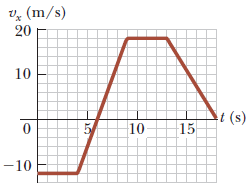# Position from velocity time graph [closed]I have taken Calculus before but unfortunately no equation is given so I have to do it the old way.

An object is at x = 0 at t = 0 and moves along the x axis according to the velocity-time graph shown below

I am asked to find the final position x of the object at t = 18s.

Here is my logic, but apparently the answer is wrong.

I find the area of each of the sections of the graph. If the graph is below the x axis it's area will be negative. I found the answer to be 97m. I got this from -44+-11+28.5+76+47.5. But this is wrong.

## closed as off-topic by John Rennie, Kyle Kanos, Daniel Griscom, Norbert Schuch, user36790 Jan 17 '16 at 2:07

This question appears to be off-topic. The users who voted to close gave this specific reason:

• "Homework-like questions should ask about a specific physics concept and show some effort to work through the problem. We want our questions to be useful to the broader community, and to future users. See our meta site for more guidance on how to edit your question to make it better" – John Rennie, Kyle Kanos, Daniel Griscom, Norbert Schuch, Community
If this question can be reworded to fit the rules in the help center, please edit the question.

• since you have no intial condition in this question, you can't solve the problem. Please specify the initial condition. – Bruce Lee Jan 16 '16 at 16:42
• @BruceLee Sorry, An object is at x = 0 at t = 0 and moves along the x axis according to the velocity-time graph shown below – frillybob Jan 16 '16 at 16:43
• Hi and welcome to the Physics SE! Please note that this is not a homework help site. Please see this Meta post on asking homework questions and this Meta post for "check my work" problems. – John Rennie Jan 16 '16 at 16:48
• PS the initial velocity is -12 not -11 – John Rennie Jan 16 '16 at 16:50
• Use $d = d_0 + v_0t + 1/2 a t^2$ where $d_0$ is the initial displacement, $v_0$ is the initial velocity, $a$ is the slope of the curve (acceleration) and $t$ is the time interval. Do the same over all four different parts of the diagram and add them up. Be careful with the signs. – Bruce Lee Jan 16 '16 at 16:52

## 1 Answer

Your approach is correct; your ability to read data from a graph is suspect (the divisions are 2 m/s each).

The initial velocity is -12 m/s, and at time t=9 s it is up to 18 m/s

That should change your answer...